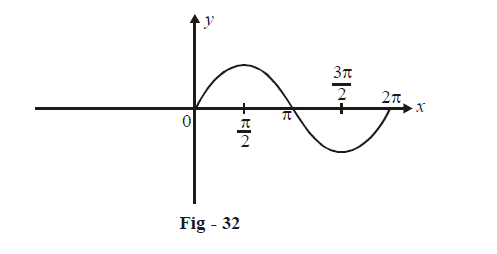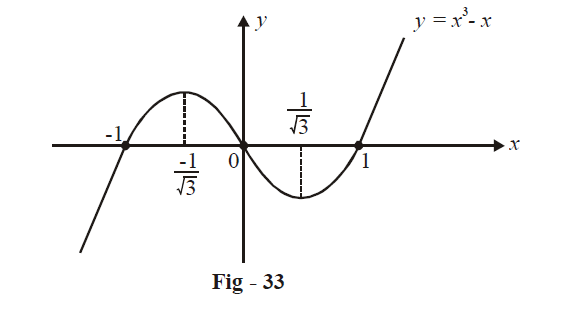# Examples on Rolles Theorem and Lagranges Theorem

## rolle's theorem examples

Example – 31

Apply Rolle’s theorem on the following functions in the indicated intervals:

(a)   $$f\left( x \right) = \sin x,\,\,x \in \left[ {0,\,\,2\pi } \right]$$      (b)   $$f\left( x \right) = {x^3} - x,\,\,x \in \left[ { - 1,\,\,1} \right]$$

Solution: (a) We know that $$f\left( x \right) = \sin x$$ is everywhere continuous and differentiable. Also,

$f\left( 0 \right) = f\left( {2\pi } \right) = 0$

$$\Rightarrow$$            From Rolle’s theorem: there exists at least one $$c \in \left( {0,2\pi } \right)$$ such that f '(c) = 0.

In fact, from the graph we see that two such c’s exist(b)  $$f\left( x \right) = {x^3} - x$$ being a polynomial function is everywhere continuous and differentiable. Also,

$f\left( { - 1} \right) = f\left( 1 \right) = 0.$

$$\Rightarrow$$            From Rolle’s theorem, there exists at least one c such that f '(c) = 0.

Again, we see that there are two such c’s given by $$f'\left( c \right) = 0$$

\begin{align} \Rightarrow \quad & 3{c^2} - 1 = 0\\\Rightarrow\quad & c = \pm \frac{1}{{\sqrt 3 }}\end{align}Example - 32

Prove that the derivative of f\left( x \right) = \left\{ {\begin{align}&{x\sin \frac{1}{x}\,\,,}&{x > 0}\\& {0\,\,\,\,,}&{x = 0}\end{align}} \right\} vanishes at an infinite number of points in \begin{align}\left( {0,\frac{1}{\pi }} \right)\end{align}

Solution: The roots of f(x) are given by

\begin{align}&\frac{1}{x} = n\pi \,\,\,;\,\,n \in \mathbb{Z} \\& \Rightarrow \quad x = \frac{1}{{n\pi }}\,\,\,;\,\,\,n \in \mathbb{Z} \qquad \ldots (i)\\\end{align}

f(x) is continuous and differentiable for all x > 0.

By Rolle’s theorem, between any two successive zeroes of f(x) will lie a zero f '(x). Since f (x) has infinite zeroes in \begin{align}\left[ {0,\frac{1}{\pi }} \right]\end{align} given by (i), f '(x) will also have an infinite number of zeroes.

Example - 33

If the function $$f:\left[ {0,4} \right] \to \mathbb{R}$$ is differentiable, then show that $${\left( {f\left( 4 \right)} \right)^2} - {\left( {f\left( 0 \right)} \right)^2} = 8f'\left( a \right)f\left( b \right)$$ for some $$a,b \in \left[ {0,4} \right].$$

Solution: Applying LMVT on f (x) in the given interval:

There exists $$a \in \left( {0,4} \right)$$ such that

\begin{align}&\qquad\quad f'\left( a \right) = \frac{{f\left( 4 \right) - f\left( 0 \right)}}{{4 - 0}}\\\\ &\Rightarrow \quad f\left( 4 \right) - f\left( 0 \right) = 4f'\left( a \right) for \;some\; a \in \left( {0,4} \right)\quad ....\ldots (i)\end{align}

Also, since f (x) is continuous and differentiable, the mean of f (0) and f (4) must be attained by f (x) at some value of x in [0, 4] (This obvious theorem is sometimes referred to as the intermediate value theorem).

That is, there exists $$b \in [0,\,4]$$ such that

\begin{align}&\qquad\;\;\; f\left( b \right) = \frac{{f\left( 4 \right) + f\left( 0 \right)}}{2}\\\\&\Rightarrow\quad f\left( 4 \right) - f\left( 0 \right) = 4f'\left( a \right) for \;some \; b \in [0\,,4] \quad........ (ii)\end{align}

Multiplying (i) and (ii), we get the desired result.

Example - 34

Using LMVT, prove that $${{e}^{x}}\ge 1+x\,\,\,for\,\,\,x\in \mathbb{R}.$$

Solution: Consider                                          $$f\left( x \right) = {e^x} - x - 1$$

$$\Rightarrow \quad f'\left( x \right) = {e^x} - 1$$

Now we apply LMVT on f (x) for the interval [0, x], assuming $$x \ge 0$$:

There exists $$c \in [0,x]$$ such that

\begin{align}f'\left( c \right) & = \frac{{f\left( x \right) - f\left( 0 \right)}}{{x - 0}}\\\\ \qquad &= \frac{{\left( {{e^x} - x - 1} \right) - \left( 0 \right)}}{x}\\\qquad & = \frac{{{e^x} - x - 1}}{x}\end{align}

Since $$f'\left( x \right)$$ is strictly increasing,

\begin{align}&\qquad\; f'\left( 0 \right) \le f'\left( c \right) \le f'\left( x \right)\\\\&\Rightarrow \qquad 0 \le \frac{{{e^x} - x - 1}}{x} \le {e^x} - 1\\\\ &\Rightarrow \qquad{e^x} \ge x + 1\,\,\,\,;x \ge 0\end{align}

Similarly, for x < 0, we apply LMVT on [x, 0] to get:

\begin{align}&\qquad\;\;{e^x} - 1 \le \frac{{{e^x} - x - 1}}{x} \le 0\\\\& \Rightarrow \qquad {e^x} \ge x + 1\,\,;x < 0\end{align}

We see that $${e^x} \ge x + 1$$  for $$x \in \mathbb{R}$$